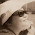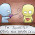## Monday, 11 February 2013

### "What's Wrong?" with these Number Line

Topic: 2 Integers, Rational & Real Numbers
Source of question: Homework 2a

Q2(a) Represent the following numbers on a number line: 5, -1/11, π, 0.9

Below are 4 number lines that have "gone wrong".
Do you know what's wrong with these number lines?

Line (1)

Line (2)

Line (3)

Line (4)

Think through before clicking at "Comments" to find out "What's Wrong".

1.Line 1:
- The numbers should be written below the line.
- The line should not stop at the 2 markings at the two ends. It should extend beyond the numbers, with an arrow pointing to the right.

Line 2:
- The line should not stop at the 2 markings at the two ends. It should extend beyond the numbers, with an arrow pointing to the right.
- Avoid using ink to draw number lines.

Line 3:
- Arrow (on the number line) pointing to the right is missing

Line 4:
- The line should not stop at the 2 markings at the two ends. It should extend beyond the numbers, with an arrow pointing to the right.
- Should use "Dots" instead of crosses.

2.The line for (1) is wrong as the intervals are equally spaced out.This shows that the number difference between two numbers are the same.Meaning that the difference between -1/11 and 0.9 is the same as the difference of 0.9 and π.The numbers should be stated below and not above the number line.
I am not so sure about line (2) but I think that the person shouldn't write the line in pen.
For line(3),
The person forgot to write the negative sign for -1/11.And the numbers that were represented should be on top and not with the other numbers
For line(4),
The person should not put a cross to represent each number.Instead,he/she should put a dot.The represented numbers should be placed on top as explained in the answer for Line(3).Furthermore,the lines should be drawn in a straight line and not free hand.

3.all the number lines are missing an arrow at the right end of the number line.
number line 1 :
The numbers should not be equally spaced out as the numbers will then be interpreted as if they have equal differences but they do not.
number line 2:
There should be more numbers in between the given numbers so as to make the placing of the given numbers more accurate. Refrain from using pens to draw the number line.
number line 4 :
crosses shouldn't be used to mark out the numbers and the work is very untidy

4.No. 1 has its numbers above the line, the markings are equally spaced out and the arrows are missing
No. 2 has no arrows
No. 3 has no arrows
No. 4 has no arows and uses crosses

5.Number 1 has its numbers being placed above the line.
Number 2 has no arrow pointing to the right and pen was used to draw the number line
Number 3 has no arrow pointing to the right direction.
Number 4 has crosses used to mark the numbers on the number line.

6.This comment has been removed by the author.

7.Number Line 1:
The numbers are equally spaced, which is a huge mistake.
Number Line 2:
There should be more numbers added to the number line.
Number Line 3:
The person had forgotten to add the negative sign to 1/11
Number Line 4:
The number line was untidy.

8.Line 1: The space should not be equally spaced. Have to extend the line at both ends. Numbers to be written below the number line. Represent the numbers using dots.

Line 2: It has no arrows pointing out and should not be using an ink pen.

Line 3: The arrow that should be pointing out is missing

Line 4: The ends was not extended. Should use dots instead of crosses.